# RD Sharma Solutions for Class 8 Maths Chapter 27 Introduction to Graphs Exercise 27.1

In Exercise 27.1 of Chapter 27, we shall see concepts related to a cartesian plane and plotting of points. Students having any doubts on the concepts of RD Sharma textbook, then quickly can refer to RD Sharma Solutions for Class 8 anytime, which is the best reference material developed by expert tutors at BYJU’S. Solutions of RD Sharma Class 8 Maths Chapter 27 are available in the pdf format, students can download the pdf from the links provided below.

## Download the Pdf of RD Sharma Solutions for Class 8 Maths Exercise 27.1 Chapter 27 Introduction to Graphs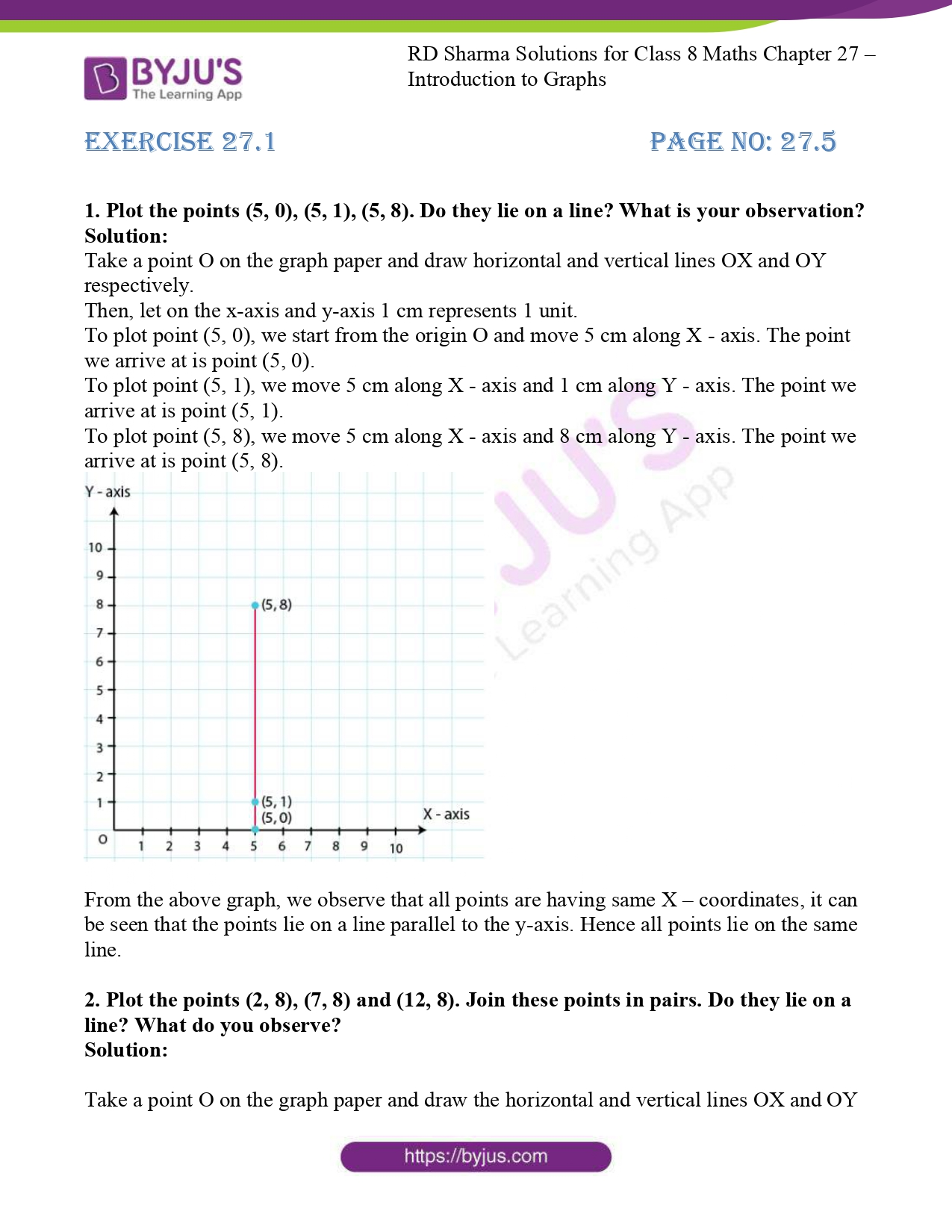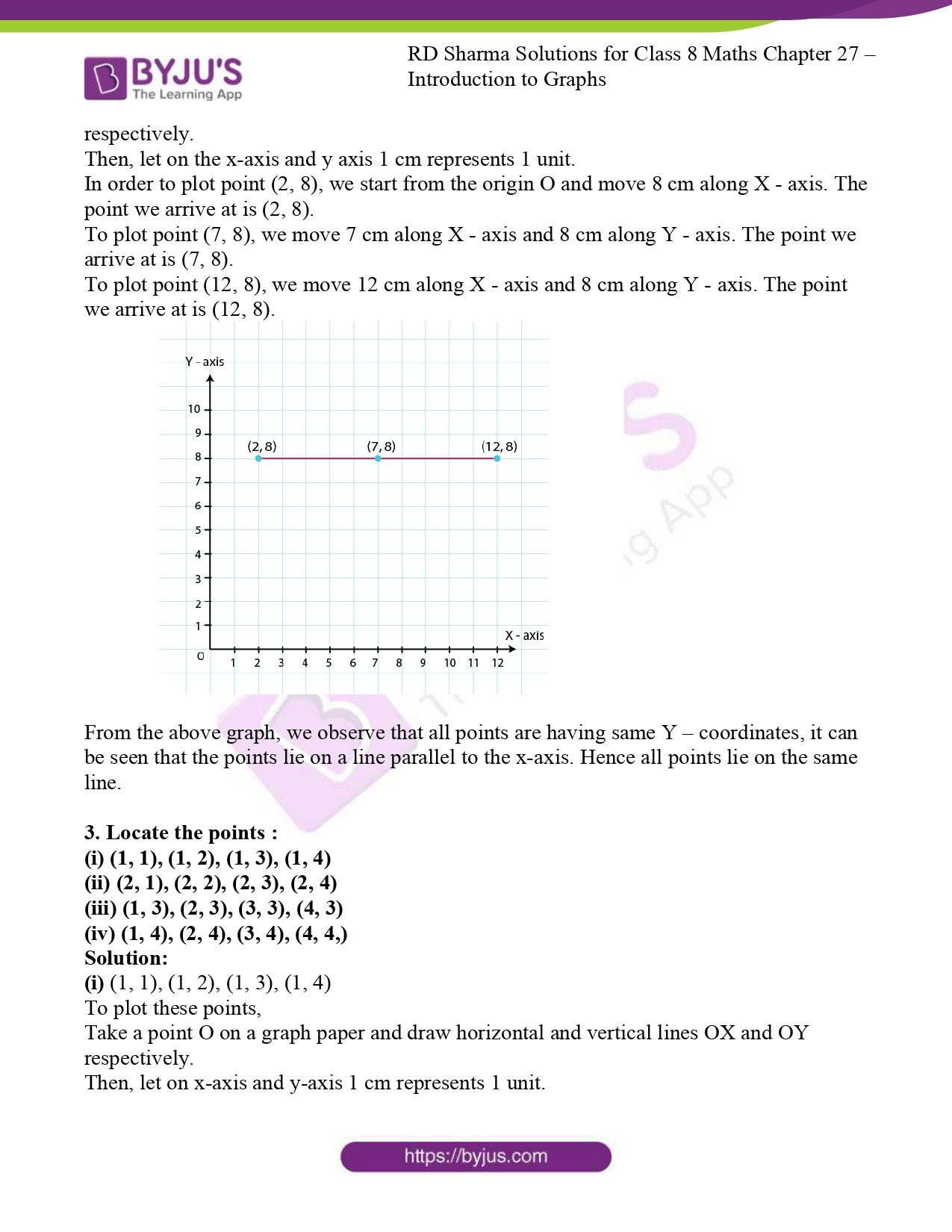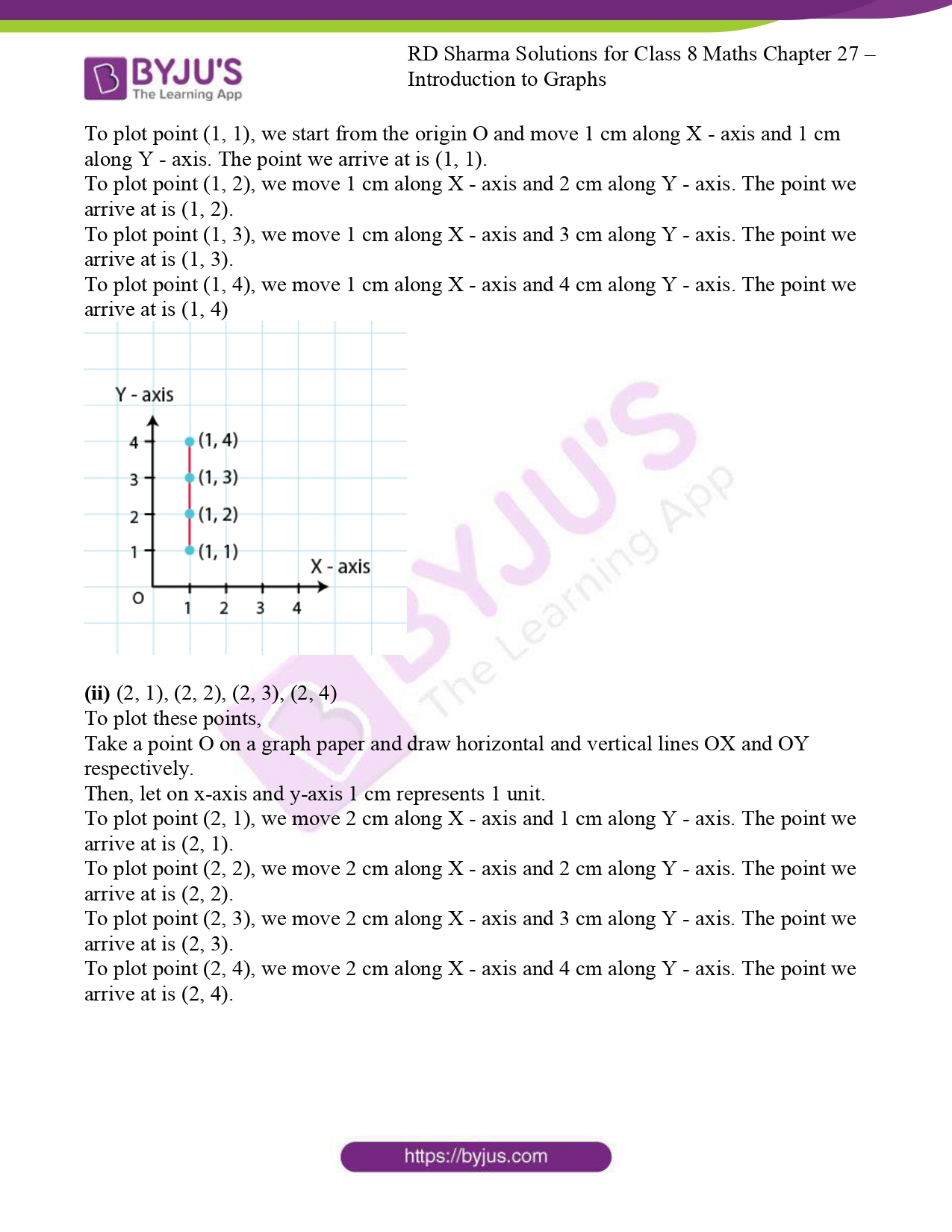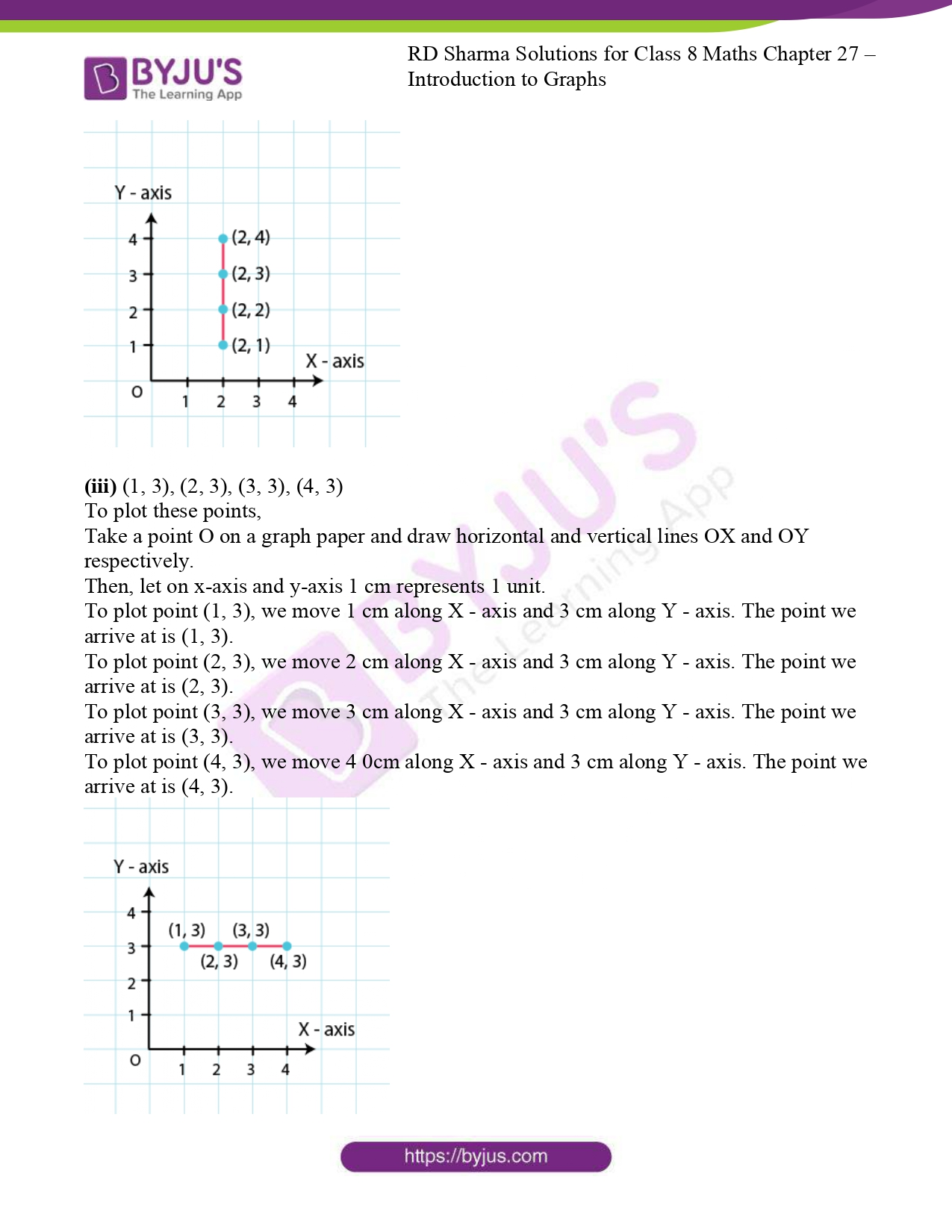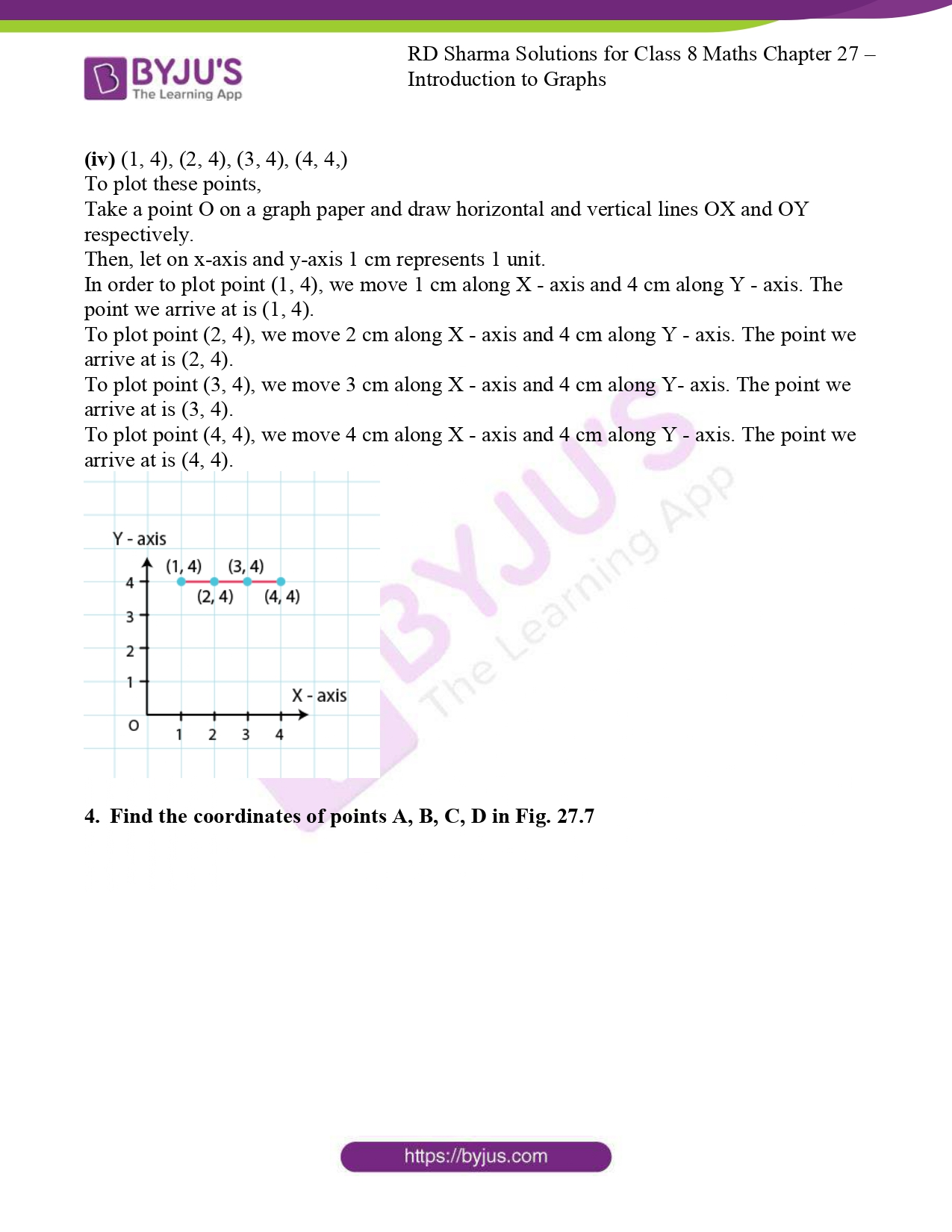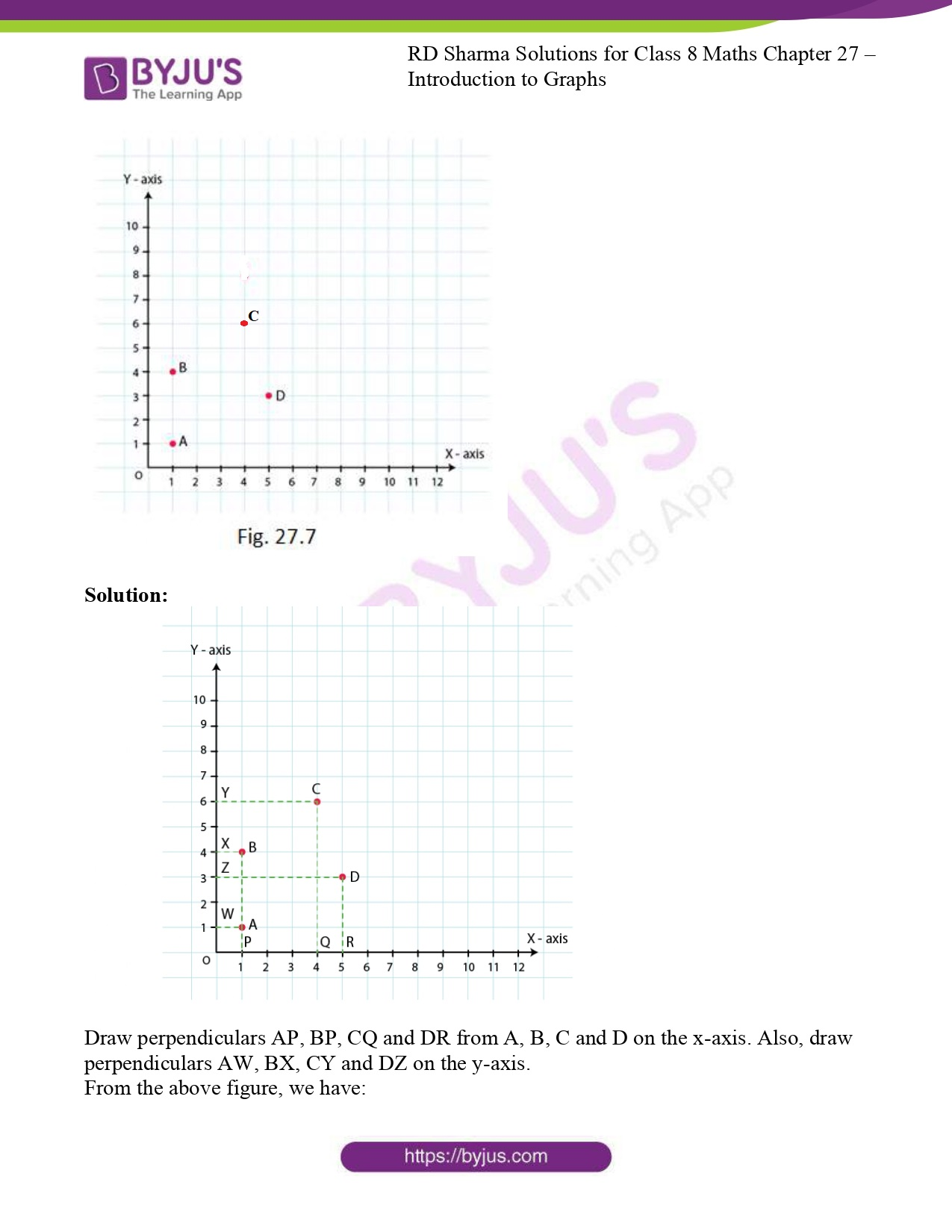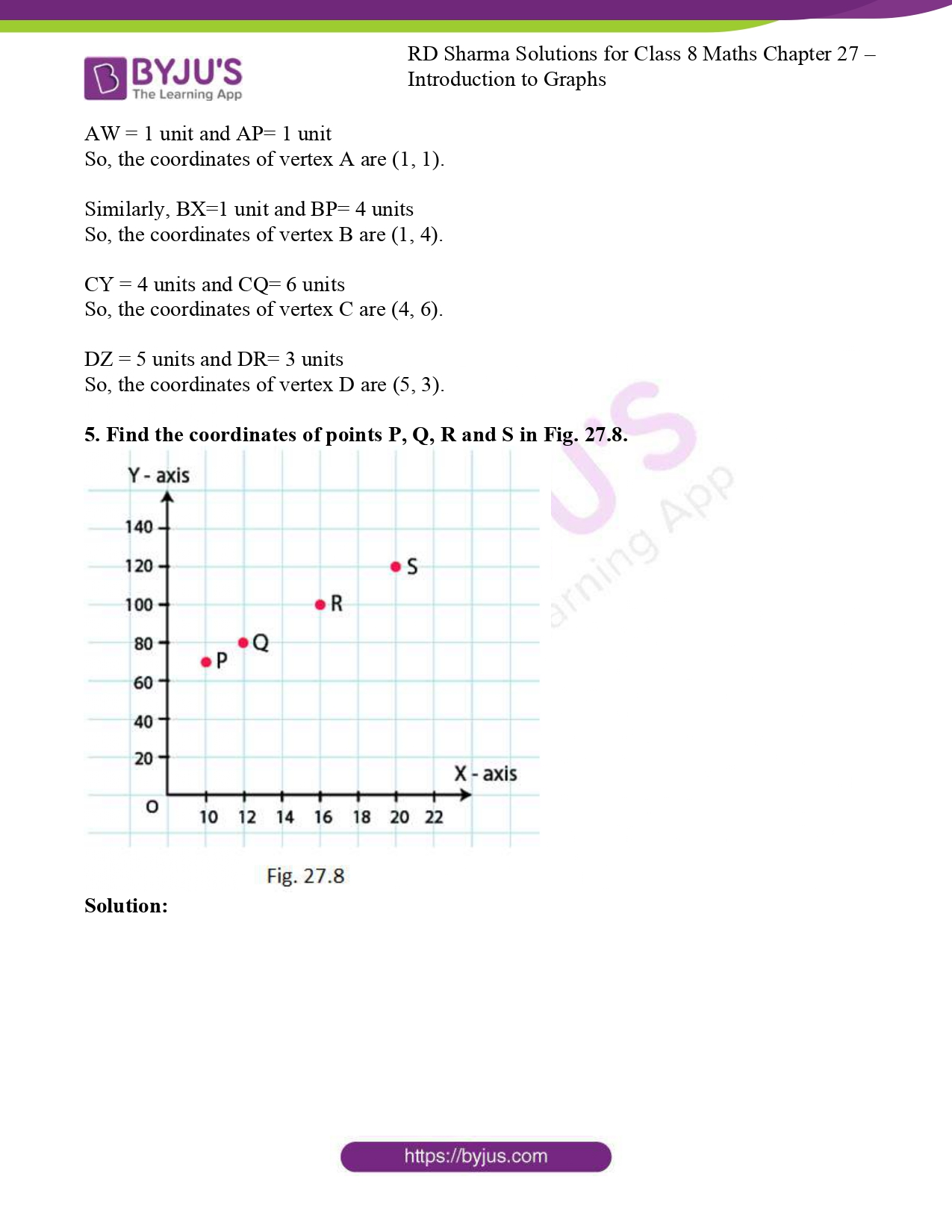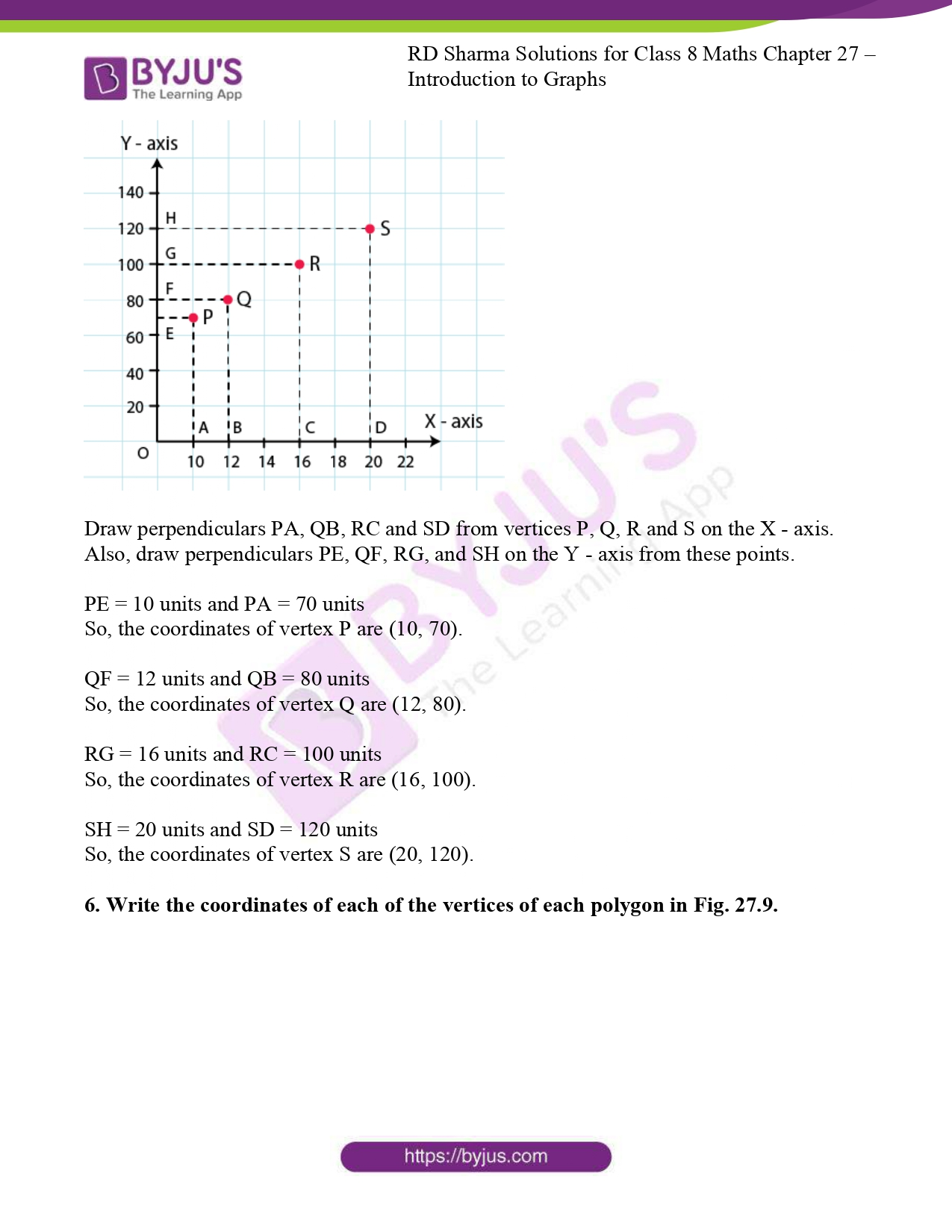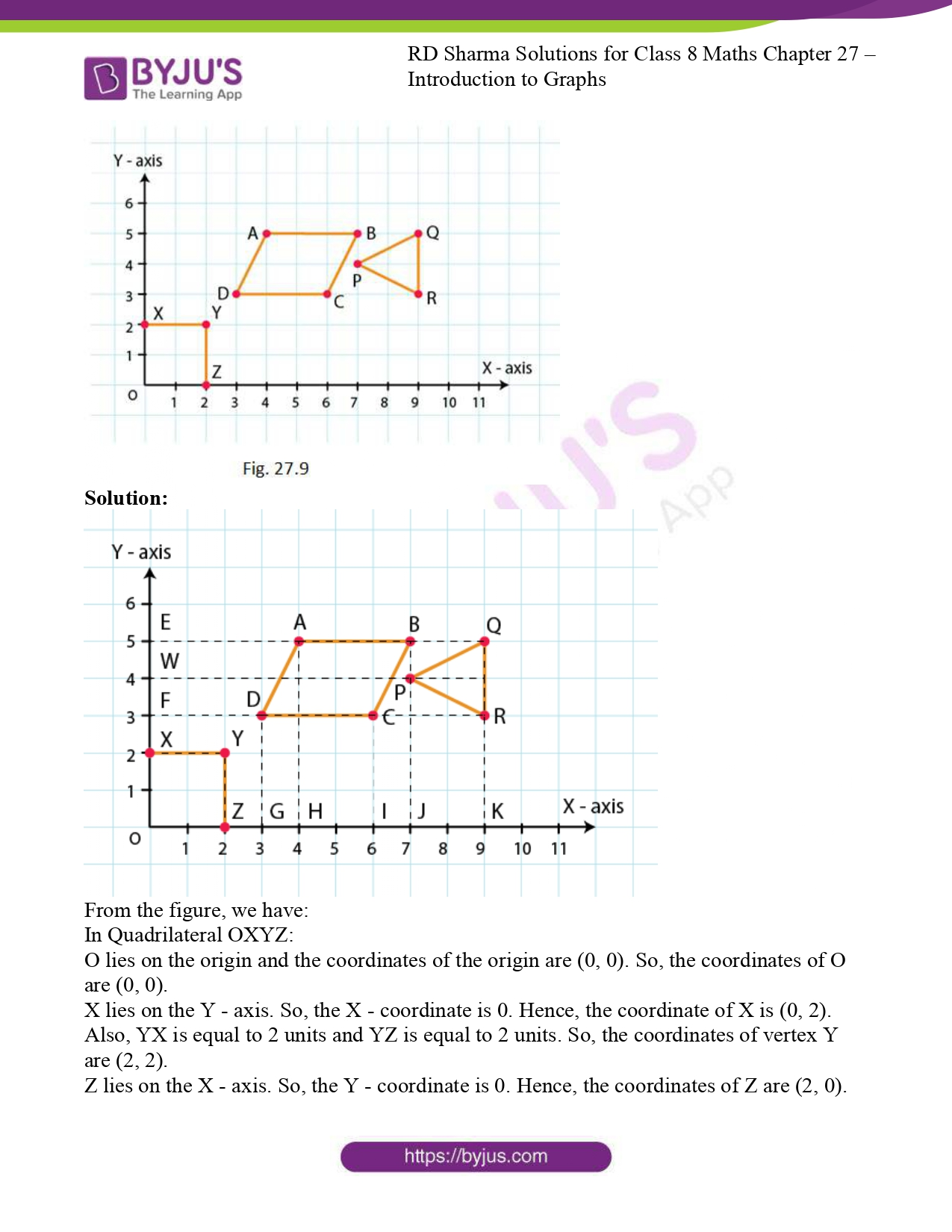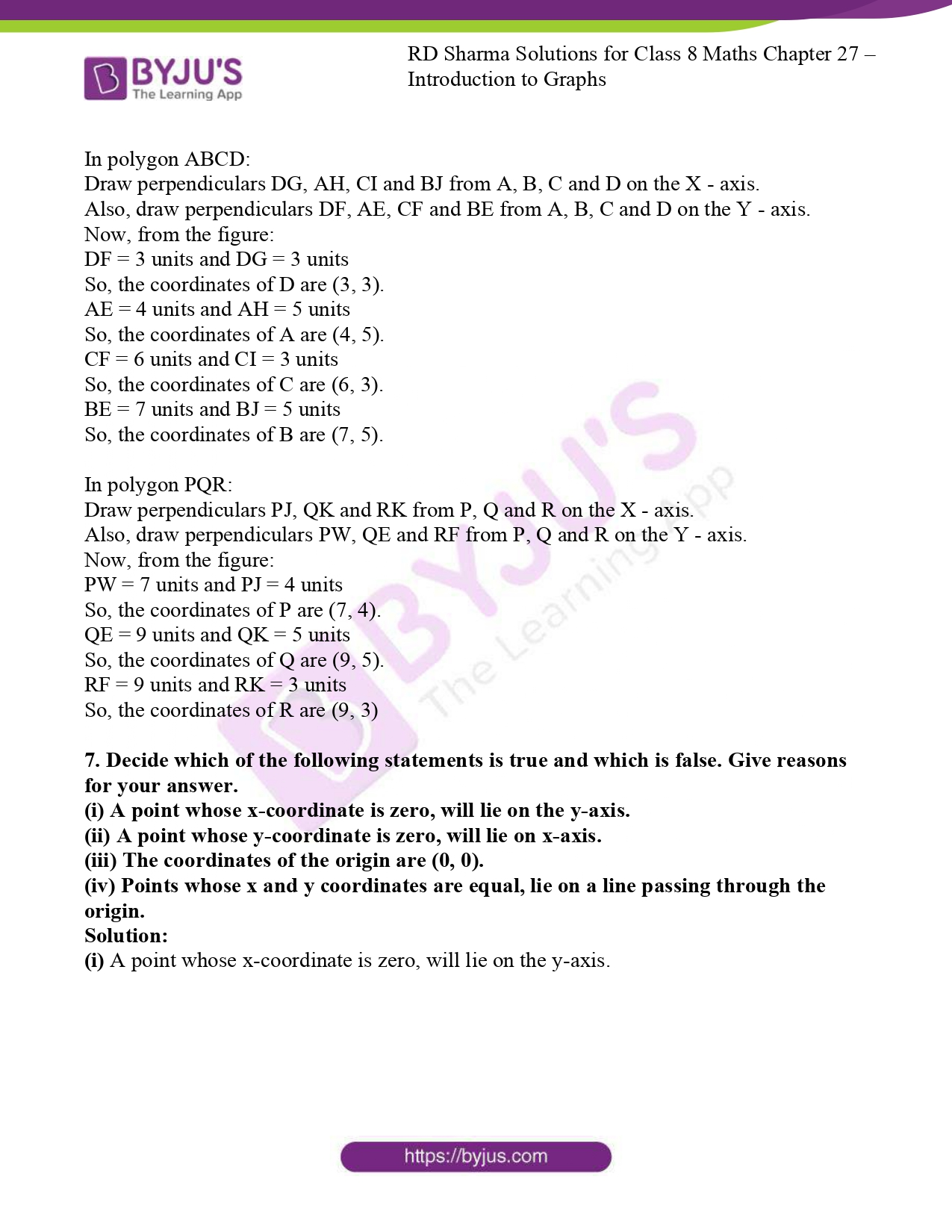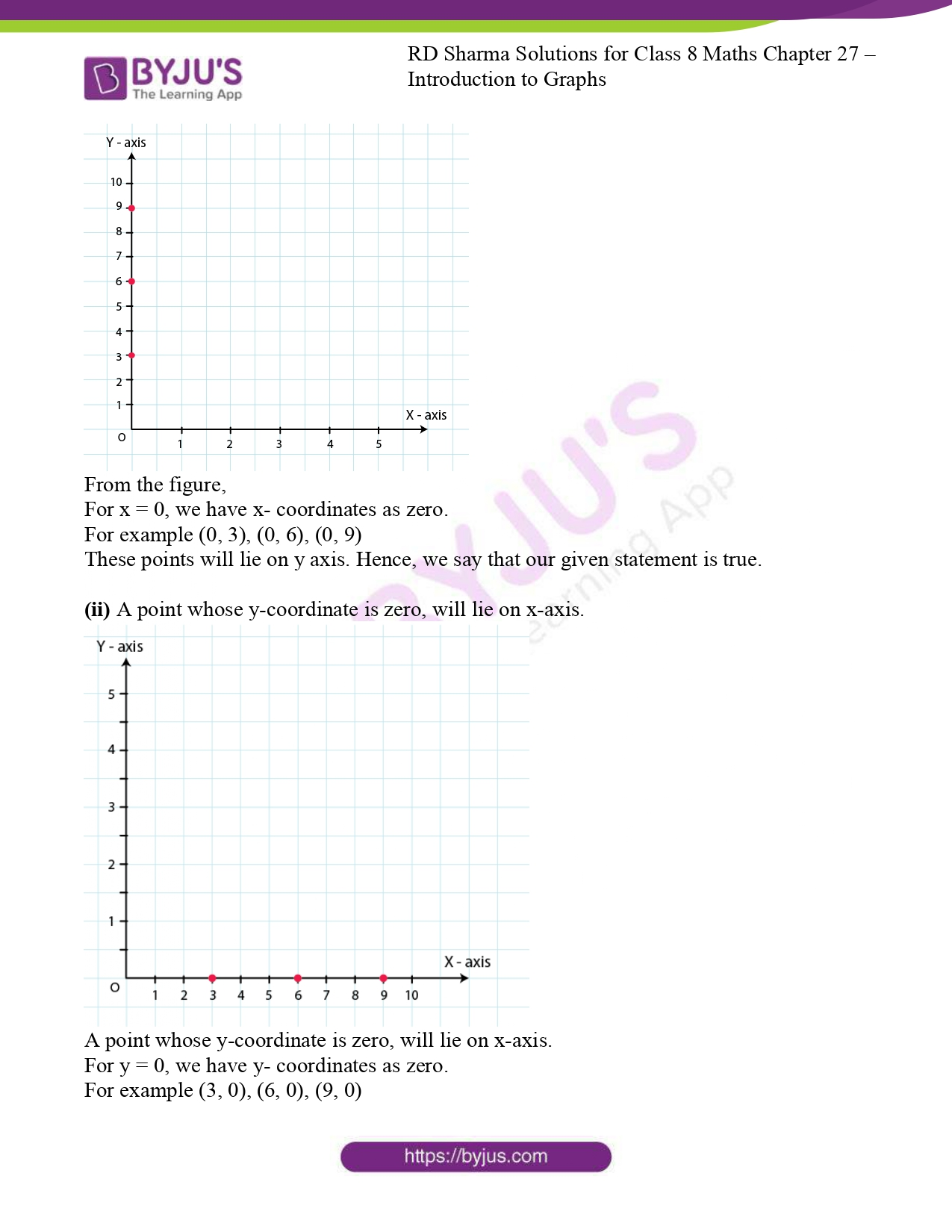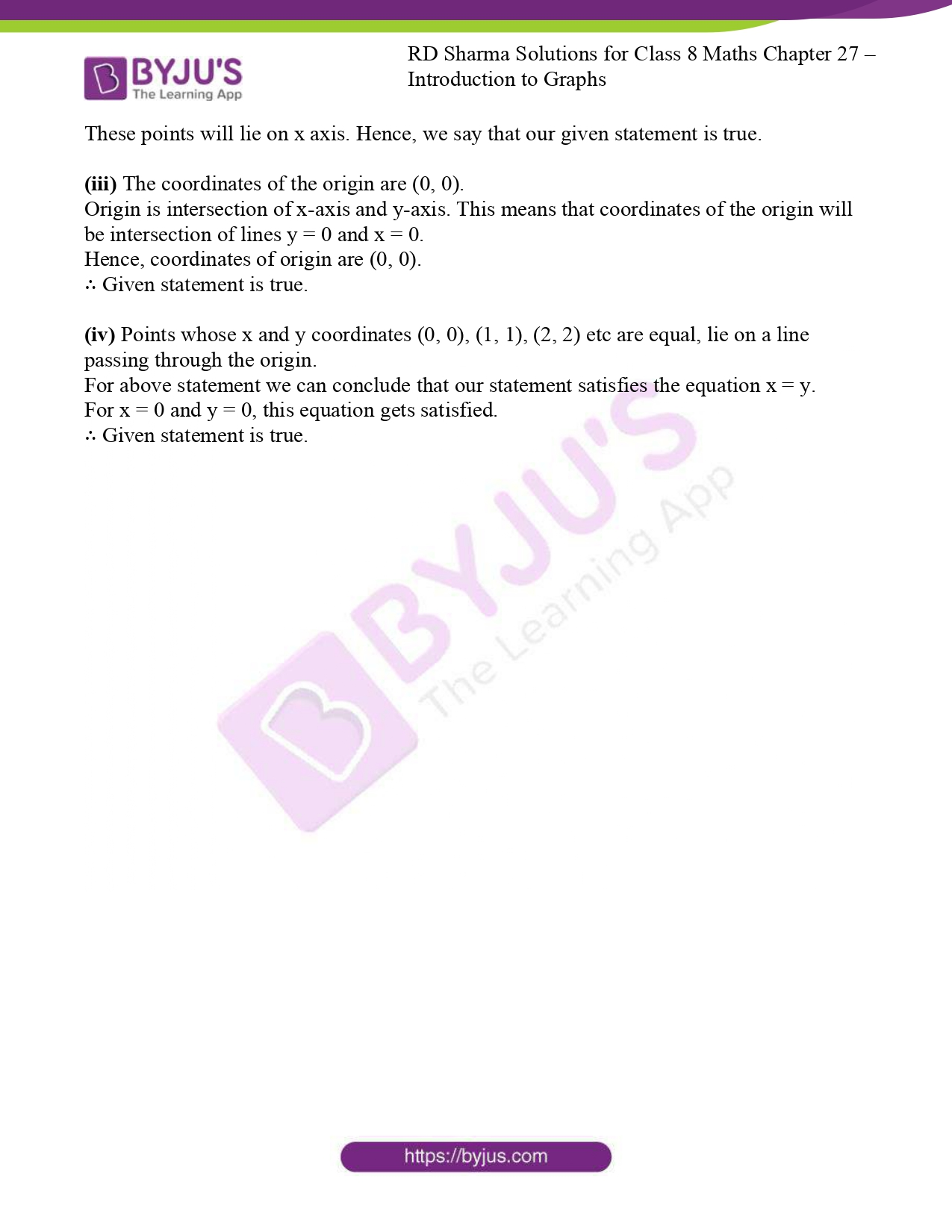### Access RD Sharma Solutions For Class 8 Maths Exercise 27.1 Chapter 27 Introduction to Graphs

1. Plot the points (5, 0), (5, 1), (5, 8). Do they lie on a line? What is your observation?

Solution:

Take a point O on the graph paper and draw horizontal and vertical lines OX and OY respectively.

Then, let on the x-axis and y-axis 1 cm represents 1 unit.

To plot point (5, 0), we start from the origin O and move 5 cm along X – axis. The point we arrive at is point (5, 0).

To plot point (5, 1), we move 5 cm along X – axis and 1 cm along Y – axis. The point we arrive at is point (5, 1).

To plot point (5, 8), we move 5 cm along X – axis and 8 cm along Y – axis. The point we arrive at is point (5, 8).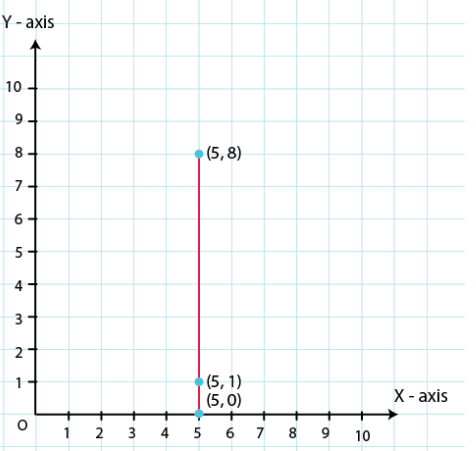From the above graph, we observe that all points are having same X – coordinates, it can be seen that the points lie on a line parallel to the y-axis. Hence all points lie on the same line.

2. Plot the points (2, 8), (7, 8) and (12, 8). Join these points in pairs. Do they lie on a line? What do you observe?

Solution:

Take a point O on the graph paper and draw the horizontal and vertical lines OX and OY respectively.

Then, let on the x-axis and y axis 1 cm represents 1 unit.

In order to plot point (2, 8), we start from the origin O and move 8 cm along X – axis. The point we arrive at is (2, 8).

To plot point (7, 8), we move 7 cm along X – axis and 8 cm along Y – axis. The point we arrive at is (7, 8).

To plot point (12, 8), we move 12 cm along X – axis and 8 cm along Y – axis. The point we arrive at is (12, 8).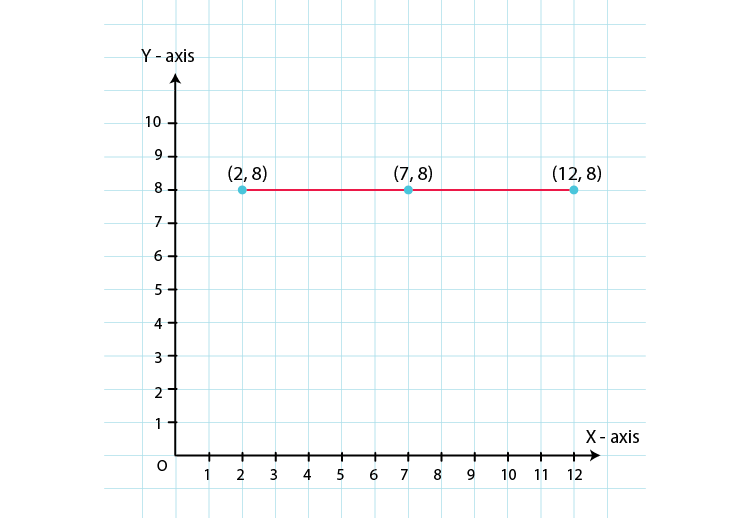From the above graph, we observe that all points are having same Y – coordinates, it can be seen that the points lie on a line parallel to the x-axis. Hence all points lie on the same line.

3. Locate the points :
(i) (1, 1), (1, 2), (1, 3), (1, 4)
(ii) (2, 1), (2, 2), (2, 3), (2, 4)
(iii) (1, 3), (2, 3), (3, 3), (4, 3)
(iv) (1, 4), (2, 4), (3, 4), (4, 4,)

Solution:

(i) (1, 1), (1, 2), (1, 3), (1, 4)

To plot these points,

Take a point O on a graph paper and draw horizontal and vertical lines OX and OY respectively.

Then, let on x-axis and y-axis 1 cm represents 1 unit.

To plot point (1, 1), we start from the origin O and move 1 cm along X – axis and 1 cm along Y – axis. The point we arrive at is (1, 1).

To plot point (1, 2), we move 1 cm along X – axis and 2 cm along Y – axis. The point we arrive at is (1, 2).

To plot point (1, 3), we move 1 cm along X – axis and 3 cm along Y – axis. The point we arrive at is (1, 3).

To plot point (1, 4), we move 1 cm along X – axis and 4 cm along Y – axis. The point we arrive at is (1, 4)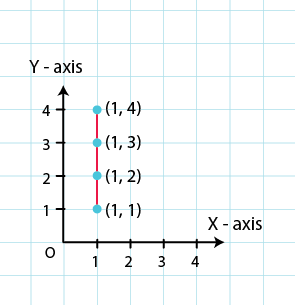(ii) (2, 1), (2, 2), (2, 3), (2, 4)

To plot these points,

Take a point O on a graph paper and draw horizontal and vertical lines OX and OY respectively.

Then, let on x-axis and y-axis 1 cm represents 1 unit.

To plot point (2, 1), we move 2 cm along X – axis and 1 cm along Y – axis. The point we arrive at is (2, 1).

To plot point (2, 2), we move 2 cm along X – axis and 2 cm along Y – axis. The point we arrive at is (2, 2).

To plot point (2, 3), we move 2 cm along X – axis and 3 cm along Y – axis. The point we arrive at is (2, 3).

To plot point (2, 4), we move 2 cm along X – axis and 4 cm along Y – axis. The point we arrive at is (2, 4).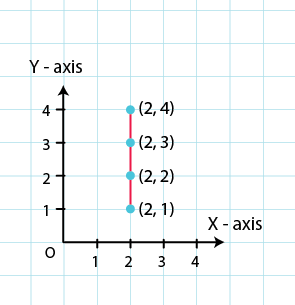(iii) (1, 3), (2, 3), (3, 3), (4, 3)

To plot these points,

Take a point O on a graph paper and draw horizontal and vertical lines OX and OY respectively.

Then, let on x-axis and y-axis 1 cm represents 1 unit.

To plot point (1, 3), we move 1 cm along X – axis and 3 cm along Y – axis. The point we arrive at is (1, 3).

To plot point (2, 3), we move 2 cm along X – axis and 3 cm along Y – axis. The point we arrive at is (2, 3).

To plot point (3, 3), we move 3 cm along X – axis and 3 cm along Y – axis. The point we arrive at is (3, 3).

To plot point (4, 3), we move 4 0cm along X – axis and 3 cm along Y – axis. The point we arrive at is (4, 3).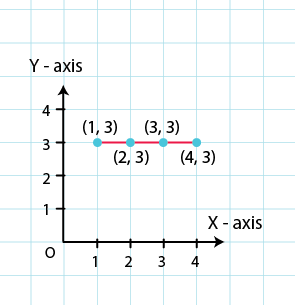(iv) (1, 4), (2, 4), (3, 4), (4, 4,)

To plot these points,

Take a point O on a graph paper and draw horizontal and vertical lines OX and OY respectively.

Then, let on x-axis and y-axis 1 cm represents 1 unit.

In order to plot point (1, 4), we move 1 cm along X – axis and 4 cm along Y – axis. The point we arrive at is (1, 4).

To plot point (2, 4), we move 2 cm along X – axis and 4 cm along Y – axis. The point we arrive at is (2, 4).

To plot point (3, 4), we move 3 cm along X – axis and 4 cm along Y- axis. The point we arrive at is (3, 4).

To plot point (4, 4), we move 4 cm along X – axis and 4 cm along Y – axis. The point we arrive at is (4, 4).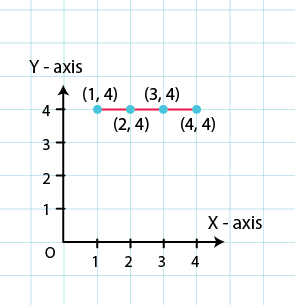4. Find the coordinates of points A, B, C, D in Fig. 27.7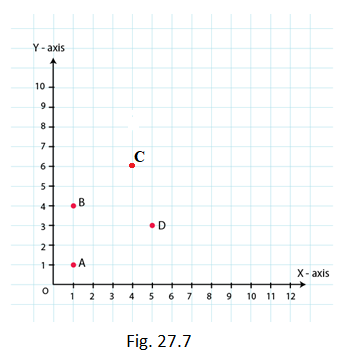Solution: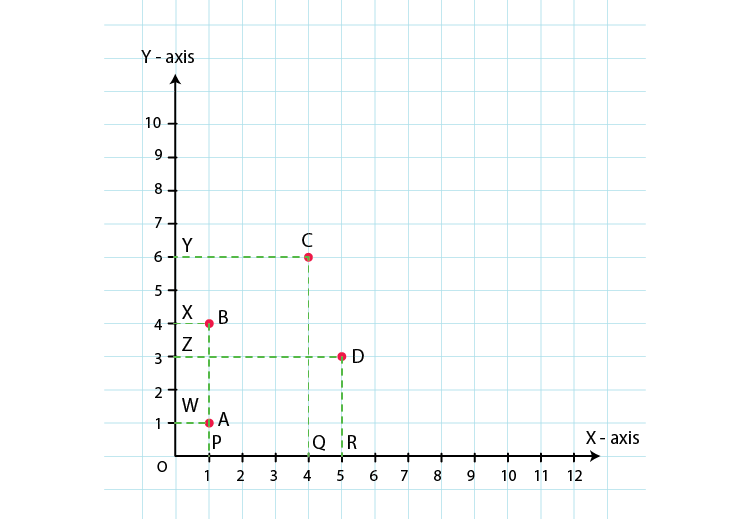Draw perpendiculars AP, BP, CQ and DR from A, B, C and D on the x-axis. Also, draw perpendiculars AW, BX, CY and DZ on the y-axis.

From the above figure, we have:

AW = 1 unit and AP= 1 unit

So, the coordinates of vertex A are (1, 1).

Similarly, BX=1 unit and BP= 4 units

So, the coordinates of vertex B are (1, 4).

CY = 4 units and CQ= 6 units

So, the coordinates of vertex C are (4, 6).

DZ = 5 units and DR= 3 units

So, the coordinates of vertex D are (5, 3).

5. Find the coordinates of points P, Q, R and S in Fig. 27.8.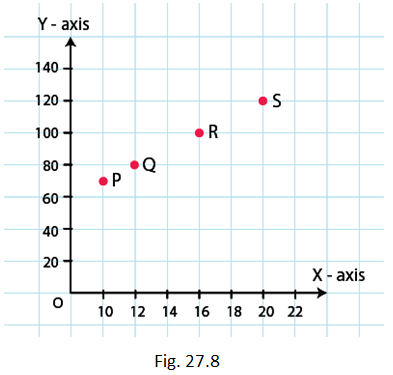Solution: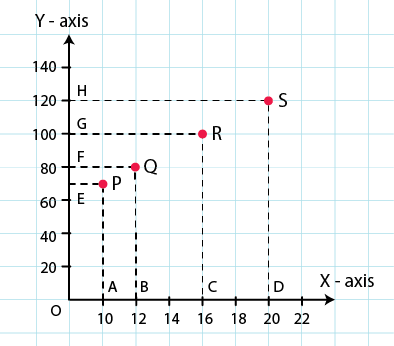Draw perpendiculars PA, QB, RC and SD from vertices P, Q, R and S on the X – axis. Also, draw perpendiculars PE, QF, RG, and SH on the Y – axis from these points.

PE = 10 units and PA = 70 units

So, the coordinates of vertex P are (10, 70).

QF = 12 units and QB = 80 units

So, the coordinates of vertex Q are (12, 80).

RG = 16 units and RC = 100 units

So, the coordinates of vertex R are (16, 100).

SH = 20 units and SD = 120 units

So, the coordinates of vertex S are (20, 120).

6. Write the coordinates of each of the vertices of each polygon in Fig. 27.9.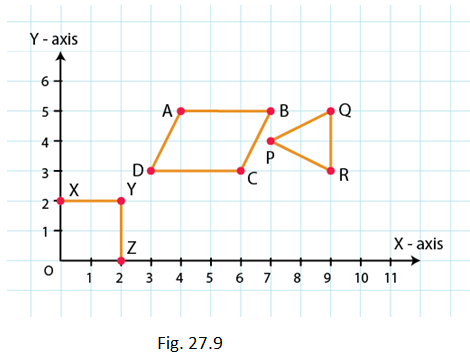Solution: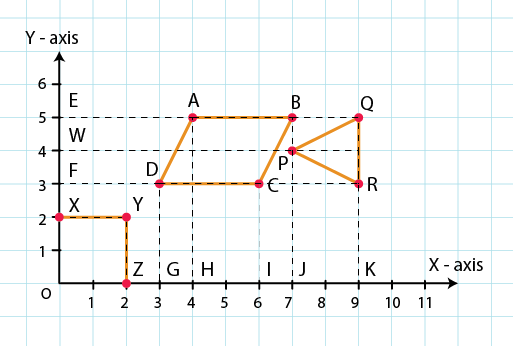From the figure, we have:

O lies on the origin and the coordinates of the origin are (0, 0). So, the coordinates of O are (0, 0).

X lies on the Y – axis. So, the X – coordinate is 0. Hence, the coordinate of X is (0, 2).

Also, YX is equal to 2 units and YZ is equal to 2 units. So, the coordinates of vertex Y are (2, 2).

Z lies on the X – axis. So, the Y – coordinate is 0. Hence, the coordinates of Z are (2, 0).

In polygon ABCD:

Draw perpendiculars DG, AH, CI and BJ from A, B, C and D on the X – axis.

Also, draw perpendiculars DF, AE, CF and BE from A, B, C and D on the Y – axis.

Now, from the figure:

DF = 3 units and DG = 3 units

So, the coordinates of D are (3, 3).

AE = 4 units and AH = 5 units

So, the coordinates of A are (4, 5).

CF = 6 units and CI = 3 units

So, the coordinates of C are (6, 3).

BE = 7 units and BJ = 5 units

So, the coordinates of B are (7, 5).

In polygon PQR:

Draw perpendiculars PJ, QK and RK from P, Q and R on the X – axis.

Also, draw perpendiculars PW, QE and RF from P, Q and R on the Y – axis.

Now, from the figure:

PW = 7 units and PJ = 4 units

So, the coordinates of P are (7, 4).

QE = 9 units and QK = 5 units

So, the coordinates of Q are (9, 5).

RF = 9 units and RK = 3 units

So, the coordinates of R are (9, 3)

7. Decide which of the following statements is true and which is false. Give reasons for your answer.
(i) A point whose x-coordinate is zero, will lie on the y-axis.
(ii) A point whose y-coordinate is zero, will lie on x-axis.
(iii) The coordinates of the origin are (0, 0).
(iv) Points whose x and y coordinates are equal, lie on a line passing through the origin.

Solution:

(i) A point whose x-coordinate is zero, will lie on the y-axis.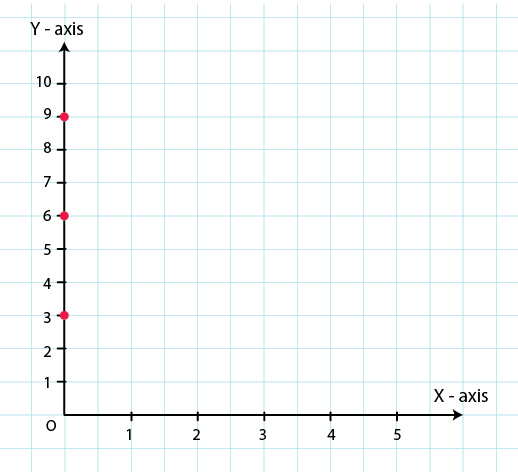From the figure,

For x = 0, we have x- coordinates as zero.

For example (0, 3), (0, 6), (0, 9)

These points will lie on y axis. Hence, we say that our given statement is true.

(ii) A point whose y-coordinate is zero, will lie on x-axis.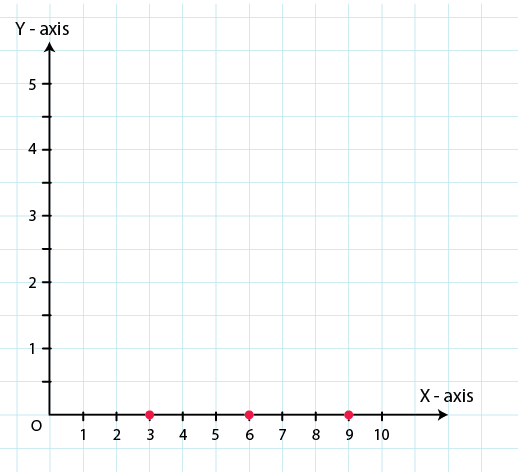A point whose y-coordinate is zero, will lie on x-axis.

For y = 0, we have y- coordinates as zero.

For example (3, 0), (6, 0), (9, 0)

These points will lie on x axis. Hence, we say that our given statement is true.

(iii) The coordinates of the origin are (0, 0).

Origin is intersection of x-axis and y-axis. This means that coordinates of the origin will be intersection of lines y = 0 and x = 0.

Hence, coordinates of origin are (0, 0).

∴ Given statement is true.

(iv) Points whose x and y coordinates (0, 0), (1, 1), (2, 2) etc are equal, lie on a line passing through the origin.

For above statement we can conclude that our statement satisfies the equation x = y.

For x = 0 and y = 0, this equation gets satisfied.

∴ Given statement is true.